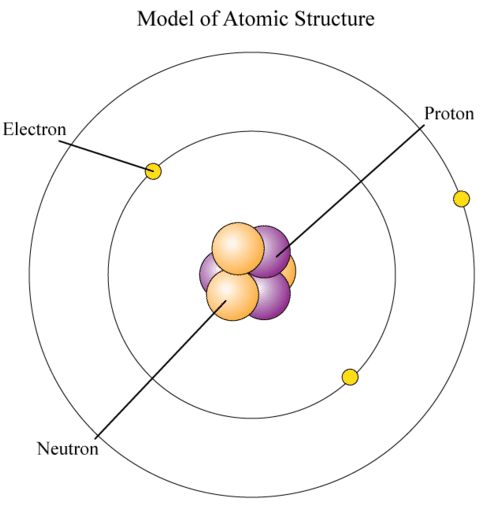# 4.8: Electrons

Watch out! Lightning is extremely dangerous. A single bolt of lightning can carry a billion volts of electricity. That's enough energy to light a 100-watt bulb for three months! As impressive as it is, lightning is nothing more than a sudden flow of extremely tiny particles. What are the particles that flow in a lightning bolt? The answer is electrons.

## What are Electrons?

Electrons are one of three main types of particles that make up atoms. The other two types are protons and neutrons. Unlike protons and neutrons, which consist of smaller, simpler particles, electrons are fundamental particles that do not consist of smaller particles. They are a type of fundamental particle called a lepton. All leptons have an electric charge of $$-1$$ or $$0$$.

## Properties of Electrons

Electrons are extremely small. The mass of an electron is only about 1/2000 the mass of a proton or neutron, so electrons contribute virtually nothing to the total mass of an atom. Electrons have an electric charge of $$-1$$, which is equal but opposite to the charge of a proton, which is $$+1$$. All atoms have the same number of electrons as protons, so the positive and negative charges cancel out, making atoms electrically neutral.

## Where are Electrons?

Unlike protons and neutrons, which are located inside the nucleus at the center of the atom, electrons are found outside the nucleus. Because opposite electric charges attract each other, negative electrons are attracted to the positive nucleus. This force of attraction keeps electrons constantly moving through the otherwise empty space around the nucleus. The figure below is a common way to represent the structure of an atom. It shows the electron as a particle orbiting the nucleus, similar to the way that planets orbit the sun. However, this is an incorrect perspective, as quantum mechanics demonstrates that electrons are more complex.## Orbitals

The atomic model in the figure above is useful for some purposes, but is too simple when it comes to the location of electrons. In reality, it's impossible to say what path an electron will follow. Instead, it is only possible to describe the chances of finding an electron in a certain region around the nucleus. The region where an electron is most likely to be is called an orbital. Each orbital can have at most two electrons. Some orbitals, called $$s$$ orbitals, are shaped like spheres, with the nucleus in the center. An $$s$$ orbital is pictured in the figure below. Where the dots are denser, the chance of finding an electron is greater. Also pictured in the figure below is a $$p$$ orbital. $$p$$ orbitals are shaped like dumbbells, with the nucleus in the pinched part of the dumbbell.Electrons are located at fixed distances from the nucleus, called energy levels. You can see the first three energy levels in the figure below. The diagram also shows the maximum possible number of electrons at each energy level.

• Electrons at lower energy levels, which are closer to the nucleus, have less energy. At the lowest energy level, which has the least energy, there is just one orbital, so this energy level has a maximum of two electrons.
• Only when a lower energy level is full are electrons added to the next lowest energy level. Electrons at higher energy levels, which are farther from the nucleus, have more energy. They also have more orbitals and greater possible numbers of electrons.
• Electrons at the outermost energy level of an atom are called valence electrons. They determine many of the properties of an element. That's because these electrons are involved in chemical reactions with other atoms. Atoms may share or transfer valence electrons. Shared electrons bind atoms together to form chemical compounds.## Summary

• Electrons are one of three main types of particles that make up the atom. They are extremely small and have an electric charge of $$-1$$. All atoms have the same number of electrons as protons.
• Negative electrons are attached to the positive nucleus. This force of attraction keeps electrons constantly moving around the nucleus. The region where an electron is most likely to be found is called an orbital.
• Electrons are located at fixed distances from the nucleus, called energy levels. Electrons at lower energy levels have less energy than electrons at higher energy levels.

## Explore More

Research the discovery of electrons at the following URLs, and then answer the questions below.

1. Who discovered electrons? When were they discovered?

2. Outline how electrons were discovered.

3. What was the significance of the discovery of electrons?

4. Where did Thomson think electrons were located in the atom? How does this differ from the modern view of electrons presented above?